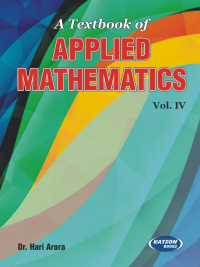•A Textbook of Applied Mathematics-IV

In Stock
ISBN 978-93-5014-684-2 Dr. Hari Arora S.K. Kataria & Sons 1st 2019 2020 2019 400 Print In Stock
Rs 350.00Rs 315.00

CONTENTS

Module-I: Iterative Techniques and Interpolation
• Solution of Algebraic and Transcendental Equation • Solution of Simultaneous Equation • Calculus of Finite Differences • Interpolation with Equal and Unequal Intervals
Module-II: Numerical Differentiation and Integration
• Numerical Differentiation and Integration • Numerical Solution at Ordinary Differential Equations
Module-III: Probability and Probability Distribution
• Probability and Probability Distribution
Module-IV: Dispersion, Curve Fitting and Principle of Least Square
• Measures of Dispersion • Curve Fitting and Principle of Least Squares
• Appendix
• Model Test Papers
• Index.

• Author : Dr. Hari Arora
• Publisher : S.K. Kataria & Sons
• Edition : 1st 2019
• Reprint : 2020
• Publishing Year : 2019
• Total Pages : 400